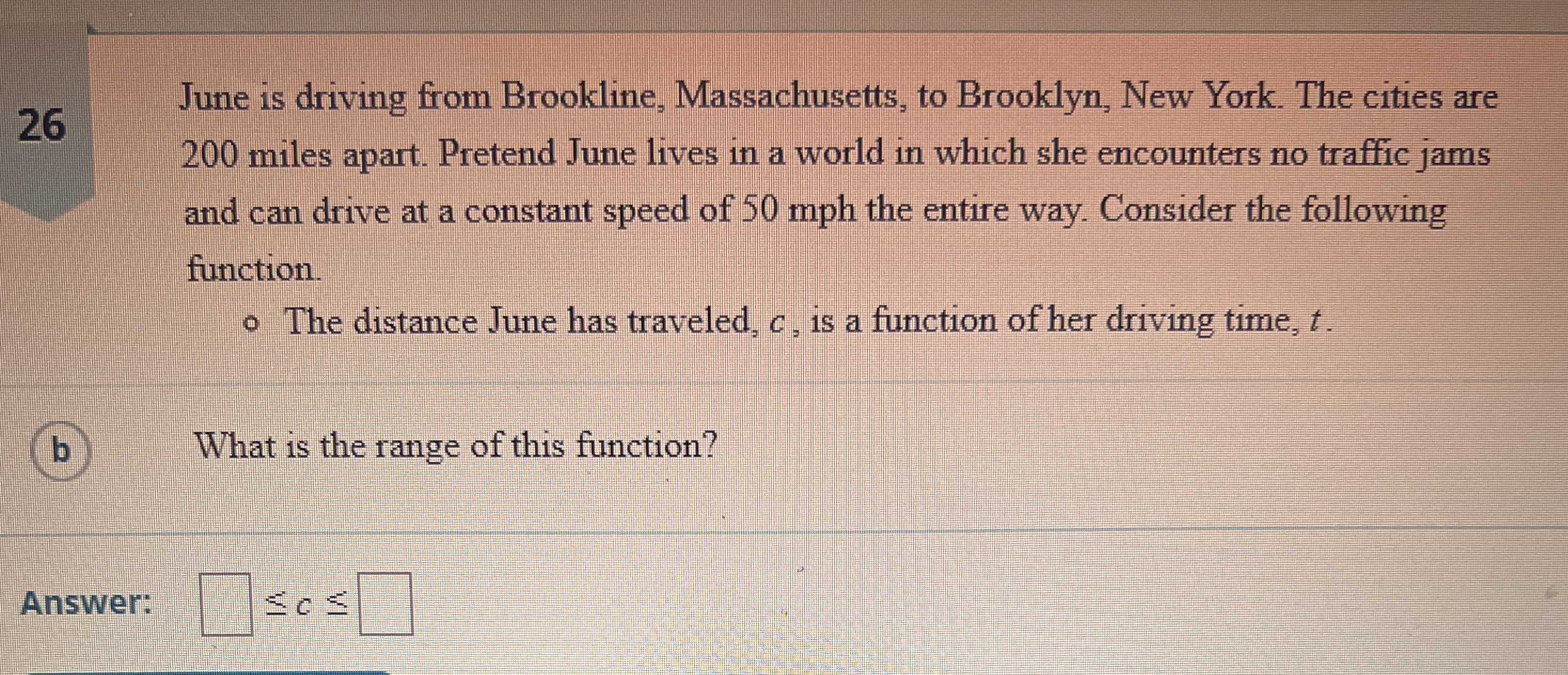### ¿Todavía tienes preguntas de matemáticas?

Pregunte a nuestros tutores expertos
Algebra
Pregunta$$26$$ June is driving from Brookline, Massachusetts, to Brooklyn, New York. The cities are

$$200$$ miles apart. Pretend June lives in a world in which she encounters no traffic jams and can drive at a constant speed of $$50$$ mph the entire way. Consider the following function. o The distance June has traveled, $$c$$ , is a function of her driving time, $$t$$ . (b) What is the range of this function? Answer: $$\square \leq c \leq \square$$

$$0\ \ \ \ \ \infty$$